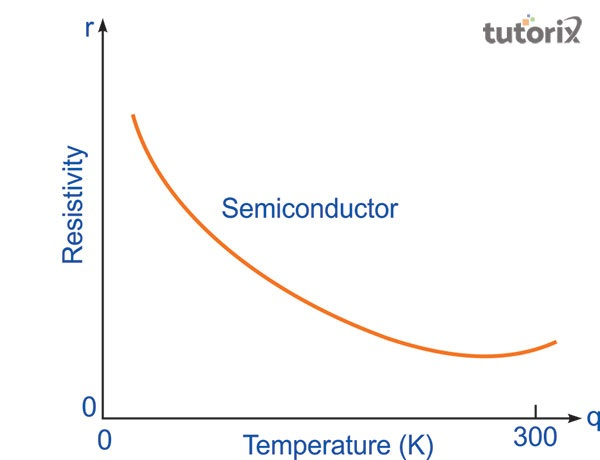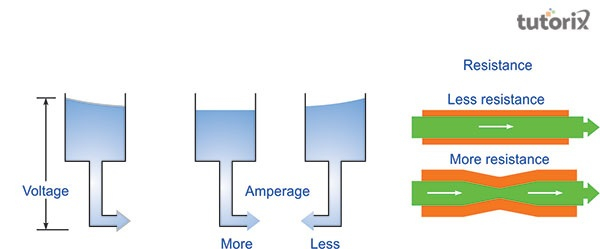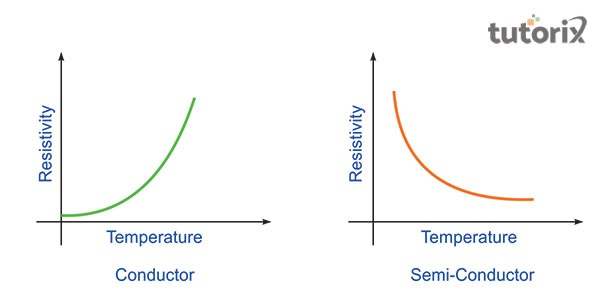# Resistivity various materials

## Introduction

Electrical resistivity is known to be the reciprocal of electrical conductivity. Resistivity of various materials is mentioned through the function of temperature as it rises with temperature due to the electron-phonon connection.

Resistivity of the material is restricted even at the ultimate zero due to the existence of small amounts of defects and impurities. Hence, it is said that conductivity and resistivity are limited and confined at ultimate zero. Resistivity's temperature coefficient is mentioned as the transformation in resistivity per unit material's resistivity per degree transformation in temperature. The electrical resistivity of various materials is the calculation of the capability of the materials to repel current flow.

Among the other types, metals possess high conductivity and low resistivity. In comparison to the metallic conductors, insulators possess high resistivity.

## What is Resistivity?

Resistivity is defined as the resistance of the area of cross-section and per unit length. Resistivity is illustrated as the material property that repelled the flow of electric current and charge. The SI unit of resistivity is termed as ohm metre $(\Omega m)$

Resistivity is tremendously helpful in contrasting distinct materials based on the ability to conduct the electric currents (Hazrol, Sapuan & Ilyas, 2018). The high resistivity of the materials entails poor conductors.Figure 1: Resistivity-temperature graph

• Resistivity is not directly proportional to the temperature. Resistivity is also described as the particular resistance of the material because the resistance minimises the thick wire. In the context of wire, resistance increases when it is thin as a result cross-sectional area is also less (Mathew & Vishnudas, 2021).

• Resistance possesses effective application in protecting the circuit from high current flow. The electrons initiate shifting from the negative to the positive electrode when acceleration is applied throughout the conductor (Stetson et al. 2019).

• Resistance often performs as a speed breaker to the flow of high current when there is a rise in current flow.

## Derivation of resistivity

The resistivity of various materials focuses on certain factors which include length, and cross-sectional area.

Length: Considering two conductors each of area of cross-section "A" and length "L". Let C be the similar potential difference applicable across two slabs' ends. The flow of current (I) across the slab is I/2. Now, resistance via every slab is

$$R\:=\:C/I\:(ohm's\:law)$$

$$Rs\:=\:C/I/2\:=\:2R$$

So, R maximises with the rise of length.

$$R\: \:\propto\:\:L....(1)$$

Cross-sectional area: Every length slab “L” possesses the area of cross-section “A/2”. Resistance through every slab based on halving conductor area is $R'\:=\:V/I/2\:=\:2R$. In this scenario, R maximises with minimisation in the area of every half slab.

$$R\:\:\propto\:\:1/A....(2)$$

Now, joining (1) and (2), it is obtained that

$$R\:\:\propto\:\:L/A$$

Let obsolete the proportionality sign, it is obtained that

$$R\:=\:p\:*\:L/A$$

Here, p is demonstrated as particular resistance or electrical resistivity.

## Relation between resistivity and conductivity

Electrical conductivity is demonstrated as the intrinsic features of the materials which are illustrated as the calculation of the electric current amount that can be carried by a material. The SI unit of electrical conductivity is demonstrated as Siemens per metre (S/m). Conductivity is the opposite of resistivity which is the calculation of how easy it is for feasible flowing of current through it.Figure 2: Electrical conductivity and resistivity

The resistivity of the material relies upon the temperature and material and is not dependent on cross-sectional area and length. Resistivity is epitomised as "p" and conductivity as "σ" (Sim et al. 2020).

Consider an instance of watering a lot of the plants during summer. If a plant is not supplied with enough water and sprinkled with a few drops, the plants may get dried and die after some time (Riwayat, Nazri & Abidin, 2018). So, with more resistance to enough water to the plants, less is the growth which means less conductivity.

## Resistivity of various materials

Conductors Resistivity Materials Silver 1.6 * 10-8 Aluminium 2.7 * 10-8 Copper 1.7 * 10-8 Iron 10 * 10-8 Alloys Nichrome 100 * 10-8 Constantin 49 * 10-8 Semiconductors Carbon 3.5 * 10-8 Germanium 0.46 Silicon 2300 Insulators Glass 1010 - 1014 Paper 1011 Hand rubber 1013 - 1016 Mica 1011 - 1015

Table : Resistivity of various materials

## Factors affecting the resistivity of various materials

The resistivity amount depends on the asset's temperature, opposing the tables of materials and usually setting the values at 20°C. Resistivity does not depend on the shape and size. Resistance to the steel conductors basically maximises with rising temperature (Saito et al. 2019). However, resistance to semiconductors like silicon and carbon generally minimises with the rising temperature.Figure 3: Comparison of resistivity of conductor and semiconductor

From the figure, it is determined that resistivity decreases with increased conductivity. On the contrary, the resistivity of the conductor rises with increasing temperature. The resistivity of semiconductors exists between that insulators and conductors as the good conductor possesses less resistivity whereas the bad conductor possesses high resistivity. According to the ohm's law, the resistivity of some material is assumed to be constant.

## Conclusion

Resistivity of materials is extremely crucial as it helps in enabling the proper materials to be utilised in the correct places in electronic and electrical elements. Materials utilised as conductors such as in general and electrical connecting wire that is required to be able to possess sensitivity level. Resistivity is expressed as ohm centimetres if the distances are calculated in centimetres. It is determined that the resistivity of various electrical materials is affected by alloying, mechanical stressing, age hardening and cool working.

## FAQs

Q1. Is resistivity directly comparable to resistance?

Ans. Resistivity is demonstrated as an innate property of a material that is directly proportional to the resistance. On the other hand, resistance is demonstrated as an external property that depends on the area of cross-section and length of the resistor.

Q2. What is the resistivity of alloys to their constituent components?

Ans. The alloy's resistivity is higher in comparison to its constituent component. It is because metal alloy has a larger resistivity as compared to corresponding metals due to lattice distortion from alloying components.

Q3. Name the substances or materials that possess good resistance.

Ans. Insulators in comparison to others possess good resistance. For instances: glass, ceramics, wood and many more.

Q4. What is the distinction between resistivity and conductivity?

Ans. Conductivity is the calculation of how the electric current easily flows. On the other hand, resistivity calculates how the material resists the electricity flow.

Updated on: 13-Oct-2022

490 Views